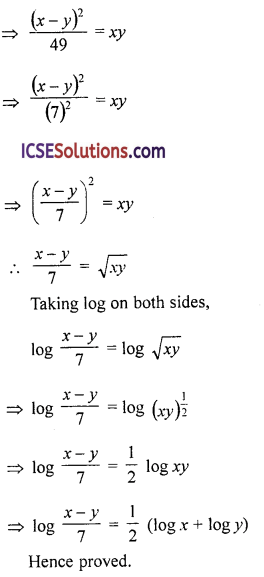Parents can use OP Malhotra Class 9 Maths Solutions Chapter 7 Logarithms Ex 7(C) to provide additional support to their children.

## S Chand Class 9 ICSE Maths Solutions Chapter 7 Logarithms Ex 7(C)

If log 2 = 0.3010, log 3 = 0.4771, log 5 = 0.6990, log 7 = 0.8451 and log 11 = find the value of the following :

Question 1.
log 6
Solution:
log 6 = log (2 x 3) = log 2 + log 3
= 0.3010 + 0.4771 = 0.7781

Question 2.
log 12
Solution:
log 12 = log (2 x 2 x 3) = log (2² x 3)
= log 2² + log 3 = 2 log 2 + log 3
= 2 (0.3010) + 0.4771 = 0.6020 + 0.4771 = 1.0791

Question 3.
log 15
Solution:
log 15 = log (3 x 5) = log 3 + log 5
= 0.4771 + 0.6990 = 1.1761

Question 4.
log 200
Solution:
log 200 = log (2³ x 5²) = log 2³ + log 5²= 3 log 2 + 2 log 5
= 3 (0.3010) + 0.6990 x 2
= 0.9030 + 1.3980 = 2.3010Question 5.
log 36
Solution:
sog 36 – log (4 x 9) = log (2² x 3²)
= log 2² + log 3² = 2 log 2 + 2 log 3
= 2 (0.3010) + 2 (0.4771)
= 0.6020 + 0.9542
= 1.5562

Question 6.
log 80
Solution:
log 80 = log (24 x 5)= log 24 + log 5
= 4 log 2 + log 5 = 4 (0.3010) + 0.6990
= 1.2040 + 0.6990 = 1.9030

Question 7.
log 2$$\frac { 1 }{ 3 }$$
Solution:
log (2$$\frac { 1 }{ 3 }$$) = log($$\frac { 7 }{ 3 }$$) = log 7 – log 3
= 0.8451 – 0.4771
= 0.3680

Question 8.
log 11³
Solution:
log (11)³ = 3 log 11
= 3 x 1.0414 = 3.1242

Question 9.
log (2$$\frac { 1 }{ 3 }$$)5
Solution:
log (2$$\frac { 1 }{ 3 }$$)5 = log ($$\frac { 7 }{ 3 }$$)5 = 5 log ($$\frac { 7 }{ 3 }$$)
= 5 [log 7 – log 3] = 5 [0.8451 – 0.4771]
= 5 (0.3680) = 1.8400

Question 10.
If log 6 = 0.7782, find the value of log 36.
Solution:
log 6 = 0.7782
log 36 = log (6)² = 2 log 6
= 2 (0.7782) = 1.5564

Question 11.
Given log10 25 = x, log10 75 = y, evaluate without using logarithmic tables, in terms of x and y.
(i) log10 3
(ii) log102
Solution: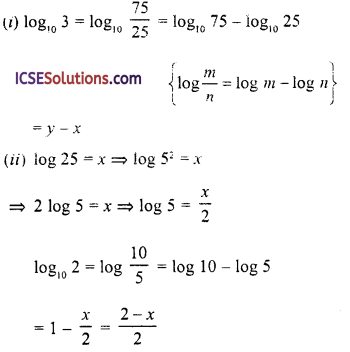Question 12.
Given log 31.87 = x, write down in terms of x.
(i) log (31.87)²
(ii) log10 0.03187
(iii) log10 $$\sqrt{31870}$$
Solution:
log 31.87 = x
(i) log (31.87)² = 2 log (31.87)
= 2x

(ii) log10 0.03187 = log10$$\frac{31.87}{1000}$$
= log10 31.87 – log10 1000
= x – 3 (log 1000 = 3)

(iii) log10 $$\sqrt{31870}$$ = log10 (31870)1/2
= $$\frac { 1 }{ 2 }$$ log10 31870 = $$\frac { 1 }{ 2 }$$ log (31.87 x 1000)
= $$\frac { 1 }{ 2 }$$ [log 31.87 + log 1000]
= $$\frac { 1 }{ 2 }$$ (x + 3) = $$\frac { x+3 }{ 2 }$$Question 13.
Solve the equation
(i) log10 (x + 1) + log10 (A – 1) = log10 11 + 2 log103
(ii) log (10x + 5) – log (x – 4) = 2
Solution:
(i) log10 (x + 1) + log10 (A – 1) = log10 11 + 2 log103
⇒ log (x + 1) (x – 1) = log (11 x 3²) (∵ 2 log10 3 = log10 3²)
⇒ log (x² – 1) = log (11 x 9) ⇒ log (x² – 1) = log 99
Comparing we get.
x² – 1 = 99 ⇒ x² = 99 + 1 = 100 = (10)²
⇒ x = 10
∴ x = 10

(ii) log (10x + 5) – log (x – 4) = 2
⇒ log $$\frac{10 x+5}{x-4}$$ = log 100 (∵ log 100 = 2)
Comparing both sides,
$$\frac{10 x+5}{x-4}=\frac{100}{1}$$
100x – 400 = 10x + 5
⇒ 100x – 10x = 5 + 400 ⇒ 90x = 405
⇒ x = $$\frac { 405 }{ 90 }$$ = $$\frac { 45 }{ 10 }$$ = 4.5
∴ x = 4.5

Question 14.
(a) Given 2 log10x + $$\frac { 1 }{ 2 }$$ log10y = 1, express y in terms of x.
(b) Express as a single logarithm :
2 log 3 – $$\frac { 1 }{ 2 }$$ log 16 + log 12
Solution: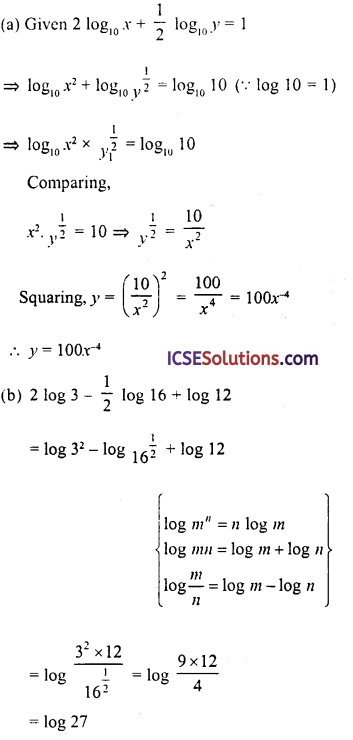Question 15.
Given that log10 y + 2log10 x = 2, express y in terms of x.
Solution:Question 16.
If a = 1 + log10 2 – log10 5, b = 2 log10 3 and c = log10 m – log10 5, find the value of m if a + b = 2c (Do not use log tables).
Solution:
a = 1 + log10 2 – log10 5 = log10 10 + log10 2 – log10 5
= log10 $$\frac { 10×2 }{ 5 }$$– = log10 4
b = 2 log10 3 = log10 3² = log10 9
c = log10 m – log10 5 = log10 $$\frac { m }{ 5 }$$
∵ a + b = 2c
∴ log10 4 + log10 9 = 2 log $$\frac { m }{ 5 }$$
⇒ log (4 × 9) = log ($$\frac { m }{ 5 }$$)² = log$$\frac { m² }{ 25 }$$
Comparing both sides,
4 x 9 = $$\frac { m² }{ 25 }$$ ⇒ m² = 4 x 9 x 25 = 900 = (30)²
∴ m = 30Question 17.
Express as a single logarithm
2 + $$\frac { 1 }{ 2 }$$ log10 9 – 2 log10 5
Solution: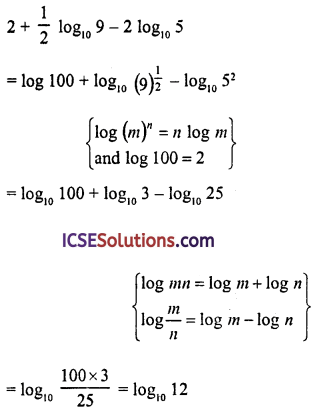Question 18.
If a = log 12, b – log 6 and c = 2 log $$\sqrt{2}$$, find
(i) a – b – c
(ii) 9a-b-c
Solution:
a = log 12, b = log 6, c = 2 log $$\sqrt{2}$$ = log
$$(\sqrt{2})^2$$ = log 2
(i) a – b – c = log 12 – log 6 – log 2
= log $$\frac { 12 }{ 6×2 }$$ = log 1 = 0 (∵ log 1 = 0)

(ii) 9a-b-c = 9° [∵ a – b – c = 0 proved in (i)]
= 1 (∵ x° = 1)

Question 19.
If x = log10 12, y = log4 2 x log10 9 and z = log10 (0.4) then find
(i) x – y – z
(ii) prove that 6x – y- z = 6
Solution:
x = log10 12, y = log4 2 x log10 9, z = log10 (0.4)
(i) x = log10 12 = log10 (2² x 3) = log 2² + log 3
= 2 log10 2 + log10 3
y = log4 2 x log109 = log42 x log10
= log4 2 x 2 log10 3 = log4 (4)$$\frac { 1 }{ 2 }$$ x 2 log10 3 (∴ loga = 1)
= log103
z = log10 0.4 = log10 $$\frac { 4 }{ 10 }$$ = log10 4 – log10 10
= log10 2² – 1 = 2 log10 2 – 1
x – y – z = 2 log10 2 + log10 3 – log10 3 – 2 log102 + 1 = 1

(ii) 6x-y-z = 61 = 6 [∵ x – y – z = 1 (proved in (i)]
Hence proved.

Question 20.
If p = log10 20 and q = log10 25, find x such that 2 log10 (x + 1) = 2p – q.
Solution:
p = log10 20 = log10 (22 x 5) = log10 2² + log10 5
= 2 log 2 + log 5
q = log10 25 = log10 (5²) = 2 log10 5
Now,
2p – q = 2 [2 log10 2 + log10 5] – 2 log10 5
= 4 log10 2 + 2 log10 5 – 2 log10 5
= 4 log10 2 = 2 log10
= 2 log10 4
2 log10(x + 1) = 2 log10 4
Comparing, we get
x + 1 = 4 ⇒ x = 4 – 1 = 3
∴ x = 3

Question 21.
Without using logarithm tables, evaluate :
3 + log10 (10-2)
Solution:
3 + log10 (10-2) = 3 + (- 2 log10 10)
= 3 – 2 log10 10 = 3 – 2 x 1
= 3 – 2 = 1 (∵ loga a = 1)
= 1Question 22.
Given log10 x = a, log10 y = b
(i) Write down 10n-1 in terms of x
(ii) Write down 102b in terms of y
(iii) If log10 P = 2a – b, express P in terms of x and y.
Solution:
(i) log10 x = a, log10y = b
∵ log10 x = a and log10 y = b
∴ 10n = x … (i)
∴ 10b = y … (ii)
Now,
(i) 10n-1 = $$\frac{10^a}{10^1}=\frac{x}{10}$$ [From (i)]
(ii) 102b = (10b)² = y² [From (ii)]
(iii) log10 P = 2a – b
⇒ log10P = 2 log10x – log10y
⇒ log10 P = log x² – log10 y
⇒ log10 P = log $$\frac { x² }{ y }$$
Comparing, we get
p = $$\frac { x² }{ y }$$

Question 23.
Simplify without using tables :
$$2 \log _{10} 5+\log _{10} 8-\frac{1}{2} \log _{10} 4$$
Solution: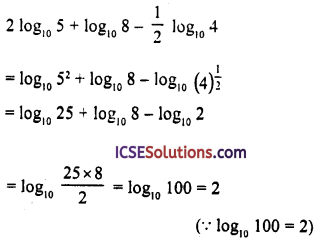Question 24.
Given that log102 = x, log10 3 = y, find
(i) log10 60
(ii) log101.2 in terms of x and y
Solution:
(i) log10 60 = log10 (2 x 3 x 10)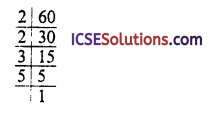= log10 2 + log10 3 + log10 10
= x + y + 1 (∵ log10 10 = 1)

(ii) log101.2 = log10 $$\frac { 12 }{ 10 }$$ = log10 12 – log10 10
= log (2² x 3) – log10 10
= 2 log 2 + log 3 – log10 10 (∵ log10 10 = 1)
= 2x + y – 1

Question 25.
Given 2 log10 x + 1 = log10 250, find
(i) x
(ii) log102x
Solution:
(i) 2 log10 x + 1 = log10 250
⇒ log10x² + 1 = log10 250
⇒ log10 x² + log10 10 = log10 250 (∵ loga a = 1)
⇒ log (x² x 10) = log10 250
Comparing both side,
10x² = 250 ⇒ x² = 25 = (5)²
∴ x = 5

(ii) log10 2x = log10 2 x 5 = log10 10 = 1

Question 26.
(a) Given that log x = m + n and log y = m – n, express the value of log10 $$\frac { 10x }{ y² }$$ in terms of in and n. (b) Solve for x : log10x = – 2.
Solution:
(a) log x = m + n, log y = m – n
log10 $$\frac{10 x}{y^2}=\log _{10} 10 x-\log _{10} y^2$$
= log10 10 + log10 x – 2 log10 y
= 1 + m + n – 2 (m – n)
= 1 + m + n – 2m + 2n
= 1 – m + 3n

(b) log10 x = – 2 = log10$$\frac { 1 }{ 100 }$$Comparing we get,
x = $$\frac { 1 }{ 100 }$$

Question 27.
If log $$\left(\frac{p+q}{3}\right)=\frac{1}{2}$$ = (log p + log q) prove that p² + q² = 7pq.
Solution:
Log $$\left(\frac{p+q}{3}\right)=\frac{1}{2}$$ = (log p + log q)
⇒ $$\log \frac{p+q}{3}=\frac{1}{2}(\log p \times q)=\log (p q)^{\frac{1}{2}}$$
Comparing we get,
$$\frac{p+q}{3}=(p q)^{\frac{1}{2}}$$
Squaring both sides,
$$\left(\frac{p+q}{3}\right)^2=p q \Rightarrow \frac{p^2+q^2+2 p q}{9}$$ = pq
⇒ P² + q² + 2 pq
⇒ p² + q² + 2pq = 9pq
⇒ p² + q² = 9pq – 2pq ⇒ 7pq
∴ p² + q² = 2pq
Hence proved.

Question 28.
If x² + y² = 51xy, prove that log $$\frac { 1 }{ 2 }$$ = $$\frac { 1 }{ 2 }$$ (log x + log y).
Solution:
x² + y² = 51xy
⇒ x² + y² – 2xy = 51 xy – 2xy
(Subtracting 2xy)
⇒ (x – y)² = 49xy